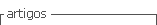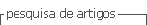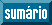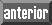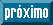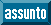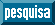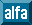## Artigo

•Citado por SciELO
•Acessos

•Citado por Google
•Similares em SciELO
•Similares em Google

## versão impressa ISSN 0012-7353

### Dyna rev.fac.nac.minas vol.85 no.205 Medellín abr./jun. 2018

#### http://dx.doi.org/10.15446/dyna.v85n205.66658

Artículos

Discrimination between the lognormal and Weibull distributions by using multiple linear regression

Discriminación entre la distribución lognormal y la distribución Weibull utilizando regresión lineal múltiple

Jesús Francisco Ortiz-Yañeza  , Manuel Román Piña-Monarrezb

a Validation Laboratory, Ted de México SA de CV, Ciudad Juárez, México. Jesus.ortiz@stoneridge.com

b Industrial and Manufacturing Department at IIT Institute, Universidad Autónoma de Ciudad Juárez, Ciudad Juárez, México. Manuel.pina@uacj.mx

Abstract

In reliability analysis, both the Weibull and the lognormal distributions are analyzed by using the observed data logarithms. While the Weibull data logarithm presents skewness, the lognormal data logarithm is symmetrical. This paper presents a method to discriminate between both distributions based on: 1) the coefficients of variation (CV), 2) the standard deviation of the data logarithms, 3) the percentile position of the mean of the data logarithm and 4) the cumulated logarithm dispersion before and after the mean. The efficiency of the proposed method is based on the fact that the ratio of the lognormal ( b1ln ) and Weibull ( b1w ) regression coefficients (slopes) b1ln/b1w efficiently represents the skew behavior. Thus, since the ratio of the lognormal ( Rln ) and Weibull ( Rw ) correlation coefficients Rln/Rw (for a fixed sample size) depends only on the b1ln/b1w ratio, then the multiple correlation coefficient R2 is used as the index to discriminate between both distributions. An application and the impact that a wrong selection has on R(t) are given also.

Key words: Weibull distribution; lognormal distribution; discrimination process; multiple linear regression; Gumbel distribution

Resumen

Palabras-clave: distribución Weibull; distribución lognormal; proceso de discriminación, regresión lineal múltiple; distribución Gumbel

1. Introduction

Because of their flexibility to model several behaviors, the Weibull and the lognormal distributions are two of the most used types of distribution in reliability. However, because the Weibull distribution is based on a non-homogeneous Poisson process, it models additive effect behavior . Similarly, because the lognormal distribution is based on a geometric Brownian motion, then it models multiplicative effect behavior . Therefore, they should not be used interchangeably. Hence, a discrimination process between both distributions is needed. In particular, the negative effect on reliability due to a wrong selection between these distributions is shown by using the stress- strength analysis, where the reliability represents all probabilities that the failure governing strength (S) exceeds the failure governing stress (s) . The stress-strength formulation is given by

In the stress-strength analysis it is assumed that time is not the cause of failure; instead, failure mechanisms are what cause the part to fail . In addition, as can be seen in eq. (1), the estimated reliability depends entirely on the selected stress and strength distributions. Thus, because a wrong selection will overestimate or underestimate reliability, a wrong selection will largely impact the analysis conclusions. To illustrate the impact of a wrong selection on reliability, following data published in Wessels has been used (, sec. 7). Table 1 shows the stress data; and Table 2 the strength data.

Finally, the stress-strength analysis for the four possible combinations between the Weibull and lognormal distributions, is presented in Table 3. The estimation of the stress-strength reliability was performed by using the eq. (40-43) given in section 7.

From Table 3, we conclude that because each combination shows a different reliability index, then the accurate discrimination between the Weibull and the lognormal distributions is an issue that must be solved. To this end, researchers have used several selection procedures. Among the oldest ones are the Chi-square, the Anderson-Darling and the Cramer-Von Mises goodness-of-fit tests . On the other hand, the most widely used methods are those based on the maximum likelihood (ML) function as they are those given in [6-10] and recently in [11-12]. In particular, the methods based on probability plot (PP) tests are in [13-15]. Those based on Kolmogorov-Smirnov (KS) test are in  and , and those based on Bayes analysis are in . The discrimination process between the Weibull and the lognormal distributions depends 1) on the relationship between the Coefficient of Variation (CV) of the observed data and their standard deviation ( σx ), 2) on the mean position of the logarithm of the data ( µx ) and 3) on the dispersion behavior before and after µx . Unfortunately, since none of the above approaches takes into account the skew behavior of the logarithm of the data, then none of them is effective in discriminating between both distributions.

Based on the fact that the Weibull data logarithm (Gumbel behavior) always presents negatively skewed behavior, the logarithm of lognormal data always presents symmetrical dispersion behavior, the b1ln/b1w ratio of the estimated lognormal and Weibull coefficients effectively discriminates between the negative and symmetrical dispersion behaviors, a method based on R2 to effectively discriminate between both distributions is offered by this paper in sec. 4. The reason for the method’s efficiency is that the R2 index for a fixed sample size (n) depends only on the b1ln/b1w ratio (see sec. 4.3). That is, because the b1ln/b1w ratio effectively discriminates between negative and symmetrical dispersion behaviors, the R2 index effectively discriminates between both distributions also.

This paper is structured as follows. Section 2 shows that the behavior of the logarithm of a Weibull variable is always negatively skewed and that the logarithm of a lognormal variable is always symmetrical. In section 3, based on the data behavior log, the characteristics that completely define whether data follow a Weibull or a lognormal distribution are given. Also, in section 3, the case where the dispersion (Sxx) contribution is not fulfilled is presented also. Section 4 shows the multiple linear regression (MLR) analysis for the Weibull and lognormal distributions. Section 5 presents 1) how via MLR, the b1ln/b1w ratio efficiently captures the Sxx dispersion behavior, and 2) that because the R2 index for a fixed n value only depends on the b1ln/b1w ratio, it captures the Sxx dispersion behavior also. The application of a stress-strength analysis is given in section 6, while Section 7 shows the effect that a wrong selection has over the reliability index. Finally, the conclusions are presented in section 8.

2. Behavior of log-Weibull and log-lognormal variables

Since the discrimination method is based on the logarithm of the Weibull or lognormal observed data and on its dispersion behavior, then in this section, we show that the Weibull data logarithm follows a Gumbel distribution and that it is always negatively skewed. Similarly, we show that the logarithm of the lognormal data follows a Normal distribution and that it is always symmetrical.

2.1. Weibull and Gumbel relationship

The Weibull distribution is given by

In eq. (2), t >0 and β and η are the Weibull shape and scale parameters respectively. On the other hand, the Gumbel distribution is given by

In eq. (3)-∞< x <∞ with x=ln(t) and μG is the location parameter and σG is the scale parameter . Thus, based on eq. (2) and eq. (3), the relation between both distributions is as follows.

Theorem: If a random variable t follows a Weibull distribution [t~W(β, η)], then its logarithm x=ln(t) follows a Gumbel distribution [ x~G(μG,σG) ] .

Proof: Let F(ln(t)) = P(ln(t) ≤ ln(T)) be the cumulative function of x = ln(t), with T representing the failure time value. Thus, in terms of x, F(ln(t)) = Pr[ln(t) ≤ x]; F(x) = Pr[t ≤ exp(x)]. Then by substituting t = exp(x), F(x) is

Finally, based on the relations between the Weibull and Gumbel parameters given by .

and by taking W=((x-µG)/σG) , eq. (4) is given by F(x) = 1-exp{-exp{(x-ln(η))·β}} which in terms of W is

from eq. (7), the reliability function is

and the density function is given by

clearly, eq. (9) in terms of W is

Since eq. (10) is as in eq. (3), we conclude that the logarithm of Weibull data follows a Gumbel distribution. On the other hand, by using the moment method  (sec. 1.3.6.6.16), the parameters of eq. (10) are given by:

where µY and σY are the mean and the standard deviation of the log data.

2.1.1. Dispersion of the Gumbel distribution

In order to show the dispersion of the log Weibull variable, several Weibull probability density functions (pdf) with fixed scale parameter η=50 and variable shape parameter β are plotted in Fig. 1. Fig. 2, corresponds to the conversion of the Weibull pdf of Fig. 1 on Gumbel pdf.

As can be seen in Fig. 2, the Gumbel distribution is always negatively skewed. Moreover, it is important to highlight that the Gumbel skew is constant at γ1= -1.13955, and as demonstrated by , it can be estimated as

On the other hand, as shown in next section, the logarithm of lognormal data follows a Normal distribution.

2.2. Lognormal and normal relationship

As it is well known, the lognormal data logarithm follows a Normal distribution . If Y~N(µ, σ2) , then X=eY (non-negative) has a lognormal distribution. Thus, because the logarithm of X yields a Normal variable (Y=ln(X)) then the lognormal distribution is given by

In eq. (13) μx and σx are the log mean and log standard deviation. Similarly, the Normal distribution is given by

Note that, although the Normal distribution is the most widely used distribution in statistics, it is rarely used as lifetime distribution. However, in reliability the Normal distribution is used as a model for ln(t), when t has a lognormal distribution.

2.2.1. Dispersion of the normal distribution

Fig. 3 represents several lognormal pdf for µx=1 and variable σx. Plotted Normal pdfs of Fig. 4 correspond to the logarithm of the lognormal pdfs plotted in Fig. 3. By comparing Fig. 3 and Fig. 4, we observe although the lognormal distribution is always positively skewed, its logarithm is always symmetrical.

Therefore, based on the log data behavior, the characteristics that completely define whether data follow a Weibull or lognormal distribution are given in next section.

3. Discrimination properties

This section presents that enough conditions are met in order to show that lognormal data follow a lognormal distribution and that Weibull data follow a Weibull distribution. Additionally, the critical characteristic to discriminate between both distributions when data follow neither a lognormal nor a Weibull distribution is given also.

3.1. Lognormal properties

In order to select the lognormal distribution as the best model to represent the data, the following characteristics have to be met. First, the coefficient of variation has to be equal to the log-standard deviation σx ( σx=CV ). Thus, because based on the mean and on the standard deviation of the observed data defined as

the CV index is given by

Then from eq. (17) clearly σx ≈ CV . Second, the log mean µx should be located at the 50th percentile. The reason is that the lognormal data logarithm follows a Normal distribution (see sec. 2.2). Third, since the total sum square (Sxx) is cumulated by the contribution before ( Sxx-- ) and after ( Sxx+ ) the mean µx is as follow

Then, due to the symmetrical behavior of the lognormal data logarithm, then in the lognormal case, the contribution before and after the mean must be equal; it is to say for the lognormal case Sxx--= Sxx+ .

Thus, because when σx ≈ CV , µx is located in the 50th percentile and Sxx--= Sxx+ , we should directly fit the lognormal model. Similarly, the characteristics to be met for the Weibull distribution are as follow:

3.2. Weibull properties

In the Weibull case, because the Weibull data logarithm follows a Gumbel distribution, and because the Gumbel distribution is always negatively skewed (See sec 2.1.1), then the following characteristics have to be met. First, the coefficient of variation should be different from the standard deviation of the data logarithm ( σx ≠ CV ). Second, the log mean µx should be located around the 36.21th percentile. Third, the contribution to Sxx before µx is always greater than the contribution after µx ; in other words, due to the negative skewness of the Gumbel distribution, in the Weibull case Sxx-->Sxx+ . Thus, because σx ≠ CV, µx is located around the 36.21th percentile and Sxx-->Sxx+ , then we should directly fit the Weibull distribution. Nonetheless, the next section will describe what happens when the above statements do not hold at all.

3.3. Weibull or lognormal distribution?

The discrimination process, when data neither completely follow a Weibull distribution nor completely follow a lognormal distribution, is based on the following facts. 1) For a Weibull shape parameter β≥2.5, the Weibull pdf is similar to the lognormal pdf . 2) For β≥2.5, the log-standard deviation σx tends to be the CV ( σx ≈ CV ), and µx tends to be located near the 50th percentile. 3) For Weibull data, regardless of the β value, the contribution before and after the mean tends to be different ( Sxx-->Sxx+ ). Now for the Normal distribution we always expect that Sxx--=Sxx+ and for the Gumbel distribution we always expect that Sxx-->Sxx+ ; thus, because from eq. (18), Sxx-- captures the skewness of the Gumbel distribution, then based on the MLR analysis, in the proposed method the product of the y vector with the Sxx-- and Sxx+ contribution is used as the critical variable to discriminate between the Weibull and the lognormal distributions. In order to show that, the linear regression analysis on which the proposed method is based must first be introduced.

4. Weibull and lognormal linear regression analysis

This section shows that by using MLR, the ratio of the slopes of the lognormal and Weibull distributions ( b1ln/b1w ) is indeed efficient to discriminate between the negative and symmetrical skew behavior. Before showing that, the MLR analysis for the Weibull and lognormal distributions will first be introduced.

4.1. Weibull linear model

The Weibull and lognormal distributions can be analyzed as a regression model of the form

The linear form of the Weibull distribution is based on the cumulative density function, given by

Thus, by applying double logarithm, its linear form is

where F(ti) is estimated by the median rank approach  given by

From eq. (21), the shape parameter β is directly given by the slope b1 , and the scale parameter η is given by

Additionally, it is necessary to note that in eq. (21)y=ln(-ln(1-F(t))) represents the behavior of the Gumbel distribution (negative skew), and that once the Weibull parameters β and η are known, the expected data can be estimated as

Clearly, from eq. (24), the ln(ti) value depends only on y. And since from the double logarithm the y values before F(t)=1-e-1=0.6321 are always negatively skewed, then in order for that data follows a Weibull distribution, its logarithm has to be negatively skewed as well. This fact implies that in the Weibull case, Sxx-->Sxx+ is always true. On the other hand, the analysis for the lognormal distribution is as follows.

4.2. Lognormal linear model

Since for the lognormal distribution the cumulative density function is given by

Then the lognormal linear relationship is given by

where µx is given by µx=-b0/b1 , and σx is given by σx=1/b1 and F(ti) is estimated as in eq. (22). On the other hand, µx and σx can respectively be estimated directly from the data as

From eq. (26) y=Φ-1(F(t)) represents the behavior of the Normal distribution (symmetrical behavior). Thus, once the lognormal parameters µx and σx are known, the expected data can be estimated from eq.(26) as follows:

On the other hand, since ln(ti) in eq. (29) follows a normal distribution, then its behavior is always symmetrical, and as a consequence of the lognormal case, the contribution to the Sxx variable is equivalent before and after μ x . In other words, in the lognormal case, Sxx -- =Sxx + . Now that it has been seen that for the Weibull distribution Sxx -- >Sxx + , and that for the lognormal distribution Sxx -- =Sxx + , let us describe the linear regression analysis to show that the ratio of the Weibull and lognormal regression coefficients efficiently represents the Sxx -- and Sxx + behavior.

4.3. Multiple linear regression analysis

In order to discriminate between the Weibull and lognormal distributions, first, the Weibull parameters of eq. (21) and the lognormal parameters of eq. (26) have to be estimated by using linear regression analysis as follows

The related multiple determination coefficient (R 2 ), is

Thus, since from eq. (30) and eq. (31), we observe that the estimated coefficients are based on the key variable Sxx, then we conclude that the regression coefficients b 0 and b 1 represent the Sxx behavior also. Based on these parameters, the proposed method is outlined in the next section.

5. Proposed method

The proposed method is based on the fact that the critical characteristic to discriminate between the Weibull and the lognormal distributions is the Sxx contribution to the log standard deviation 𝜎 𝑥 . Thus, in order to present the steps of the proposed method to discriminate between the Weibull and lognormal distributions, it is necessary first to show that via MLR, the regression coefficients (slopes) b 1ln /b 1w ratio completely incorporates the negative skew and the symmetrical behavior of the observed data, and that the multiple linear regression coefficient R 2 completely depends on the b 1ln /b 1w ratio.

5.1. The ratio b 1ln /b 1w efficiently capture the Sxx behavior

The analysis for the Weibull and lognormal distributions is given below.

5.1.1. Weibull analysis

In order to show that the regression coefficients (slopes) b 1ln /b 1w ratio completely incorporates the skew behavior of the Weibull distribution represented by Sxx, it is necessary to first show that based on the b 1ln /b 1w ratio given by

For the Weibull distribution, Sxy w >Sxy ln . To observe this, it should be remembered that because the Weibull response variable y w given by y w =ln[-ln(1-F(t))] is higher weighted in the initial values (lower percentiles), and because for Weibull data, Sxx - tends to be greater than Sxx +, then the impact of Sxx -- over Sxy w given by Sxy w =y w (x-µ) is higher in the initial values. As should be noted, this fact implies that when data follows a Weibull distribution, the difference between Sxy w and Sxy ln tends to be higher. Likewise, from eq. (33), this fact implies that for Weibull data the b 1ln /b 1w ratio or Sxy ln /Sxy w decreases.

5.1.2. Lognormal analysis

In the lognormal case, because the lognormal response variable y ln , given by y ln = Φ -1 (F(t)), is symmetrical around the 50th percentile, then for lognormal data Sxx - it tends to be Sxx + (see sec 3.1). As a consequence, the impact of Sxx -- on Sxy ln =y ln (x-µ) is lower than that of the Weibull distribution. This fact implies that for lognormal data, the difference between Sxy w and Sxy ln tends to be lower than when data is Weibull. As a result of this lower impact, when data is lognormal in eq. (33), the b 1ln /b 1w ratio or its equivalent Sxy ln /Sxy w increases.

Thus, because based on the Sxx behavior, for Weibull data the b 1ln /b 1w ratio decreases, and for lognormal, data it increases, then we conclude that because Sxy=y(x-µ) clearly captures the behavior of Sxx, then the b 1ln /b 1w ratio efficiently captures the behavior of Sxx also.

Now it will be shown that because the R 2 index depends only on the b 1ln /b 1w ratio, then it also captures the behavior of Sxx. Consequently, the R 2 index can also be used to discriminate between the Weibull and the lognormal distributions.

5.2. The R 2 index is completely defined by the b 1ln /b 1w ratio

In order to show that the R 2 index is completely defined for the b 1ln /b 1w ratio, it will be first be noted that based on eq. (32), the relationship between b 1w parameter and the Weibull R w index and the relationship between the b 1ln parameter and the lognormal R ln index can be formulated by the following relation

Secondly, in doing this it should be observed that by taking away Sxy=b 1 Sxx from eq. (31), and by replacing it in eq. (34), b 1 is directly related with σ x , σ y and R 2 , as follows

where

Thus, from eq. (35), the Weibull b 1w and R w values are related with the lognormal b 1ln and R ln values as follows

Next, it will be shown that because the R ln /R w ratio depends only on the b 1ln /b 1w ratio, then the R 2 index can be used to efficiently discriminate between the Weibull and the lognormal distributions. Having done this, it should also be noted from eq. (38) and eq. (39) that σ x is the standard deviation of the data logarithm, and that it is the same for both distributions. This fact (σ x = σ x ) implies from eq. (38) and eq. (39) that

Therefore, based in eq. (40), the relationship between the R ln and the R w indices is given by

And because the σ y w y ln ratio is constant in the analysis, we conclude that the R ln /R w ratio depends only on the b 1ln /b 1w ratio. Consequently, the R 2 index is efficient to discriminate between the Weibull and the lognormal distributions. Additionally, it is important to highlight that the σ yw yln ratio in eq. (41) is constant also, and that this is so because σ y defined in eq. (36) depends only on the sample size n. Thus, once n is known (or selected, see  eq. (13)), σ y is constant.

5.3. Steps of the proposed method

Because based on the observed data, the R2 index efficiently represents the Sxx behavior, then based on the observed data, the steps of the proposed method to discriminate between the Weibull and the lognormal distributions are as follows.

By using the Weibull y vector defined in eq. (21) (or the lognormal y vector defined in eq. (26)) and the observed data logarithm (ln(t)=x), the Weibull (or lognormal) correlation is estimated as Sxy=∑yi(xix).

• From the logarithm of the observed data, estimate the variance of x as Sxx=∑(x i x ) 2 .

• By using the Weibull (or lognormal) Sxy value from step 1 and the Sxx value from step 2 into eq. (31), estimate the Weibull (or lognormal) slope b 1 coefficient.

• By using the Weibull y vector defined in eq. (21) (or the lognormal y vector defined in eq. (26)), estimate the Weibull (or lognormal) variance of y as Syy=∑(y i y ) 2 .

• By using the Weibull (or lognormal) slope b 1 coefficient from step 3, Weibull (or lognormal) Sxy value from step 1 and the Weibull (or lognormal) Syy value from step 4 into eq. (32), estimate the Weibull (or lognormal) coefficient R 2 .

• Compare the Weibull and the lognormal R 2 indices, select the distribution with higher R 2 value. If R w 2 >R ln 2 select Weibull distribution; otherwise select lognormal distribution.

6. An application

The efficiency of the R 2 index to discriminate between the Weibull and the lognormal distribution is shown in a stress-strength analysis by using data in section 1. Table 1 Data corresponds to the stress load in a machine that uses a plunger to press a shaft into a bushing. Table 2 Data corresponds to the strength of the plunger when it is subjected to compression loads . Thus, the selection of the stress distribution by using the proposed method is as follows.

6.1. Stress data analysis

From the stress observed data shown in Table 4, we note that because 1) the σ x ≈CV (σ x =CV=0.0055), 2), µ x is located near the 50th percentile, and 3) Sxx -- = 53% ≈ Sxx + = 47%. Then, from section 3.1, it is reasonable to expect that the lognormal distribution represents the data.

The above statement is verified by applying the proposed method to the Table 1 data. The required values Sxy, Sxx and Syy to apply the method are estimated by applying the MLR analysis to the stress data. The values for the Weibull and the lognormal distributions are given in Table 4.

Thus, by using data of Table 4, the lognormal analysis is as follows. From step 1, Sxy ln =7.1714 (column 9). From step 2, Sxx=1.3412 (column 10). From step 3, and eq. (31), b 1ln =5.3471. From step 4, Syy ln =39.4812 (column 13). Therefore, from step 5, and eq. (32), R ln 2 =0.9712.

Similarly, by applying the proposed method to the Weibull distribution, we have from step 1, Sxy w =8.8996 (column 8). From step 2, Sxx, as in the lognormal case, is also Sxx=1.3412 (column 10). From step 3, and eq. (31), b 1w =6.6357. From step 4, Syy w =61.9775 (column 12). Therefore, from step 5, and eq. (32), R w 2 =0.9528.

Finally, as expected, by comparing the Weibull and lognormal R 2 indices, in step 6, we have that R ln 2 =0.9712>R w 2 =0.9528. Thus, we conclude that the failure governing the stress distribution is the lognormal distribution. On the other hand, the selection of the strength distribution by using the proposed method is as follows.

6.2. Strength data analysis

The strength data is given in Table 5. From this data, we note that while 1) the σ x ≈CV (σ x =CV=0.0077) and 2) the µ x is located near the 50th percentile, 3) the Sxx -- contribution is greater than the Sxx + contribution Sxx -- =61%> Sxx + =39%. Thus, because from section 3.3 the characteristics of the lognormal distribution are not completely met, then we conclude that data can be better represented by the Weibull distribution. However, the estimation of the R 2 index is necessary. When doing this, the values of Sxy, Sxx and Syy are estimated by using an MLR analysis of the strength data. The MLR analyses for the Weibull and the lognormal distributions are summarized in Table 5.

By using Table 5 data and by applying the proposed method for the lognormal distribution, we have that, from step 1, Sxy ln =1.9402 (column 9). From step 2, Sxx=0.3298 (column 10). From step 3, and eq. (31), b 1ln =5.8820. And from step 4, Syy ln =12.2451 (column 13). Therefore, from step 5, and eq. (32) R 2 =0.9319.

Similarly, by applying the proposed method for the Weibull distribution, we have that, from step 1, Sxy w =2.4320 (column 8). From step 2, Sxx as in the lognormal case, is Sxx=0.3298 (column 10). From step 3, and eq. (31), b 1w =7.3730. And from step 4, Syy w =18.5330 (column 12). Therefore, from step 5, and eq. (32), R w 2 =0.9675.

Finally, by comparing the R 2 indices as in step 6, we have that R w 2 =0.9675>R ln 2 =0.9319. Thus, the failure governing the strength distribution is the Weibull distribution.

As a summary, because the stress data follows a lognormal distribution and the strength data follows a Weibull distribution, then for the stress-strength analysis the lognormal-Weibull combination has to be used. Therefore, from Table 3, the corresponding lognormal-Weibull reliability is R(t)=0.9860. Finally, the effect that a wrong selection of the distribution has over the estimated reliability is given in Table 3. Although the reliability values given in Table 3 were estimated by using the Weibull++ software, the next section provides the formulas to estimate such values.

7. Stress-Strength reliability

The stress-strength reliability values of Table 3 were estimated as follow. For the lognormal-lognormal stress-strength, the formulation given in eq. (42) was used

For the lognormal-Weibull stress-strength, the formulation given in eq. (43) was used

For the Weibull-lognormal stress-strength, the formulation given in eq. (44) was used

Finally, for the Weibull-Weibull stress-strength, the formulation given in eq. (45) was used

Where

8. Conclusions

The reliability analysis for the Weibull and the lognormal distributions is performed by using the data logarithm. For the Weibull distribution, the logarithm data is negatively skewed. For the lognormal distribution, the logarithm data is symmetrical. Because for the Weibull distribution, the contribution to the variance before the mean is always greater than the contribution after the mean [Sxx -- >Sxx + ], then this behavior is used to discriminate between the Weibull and the lognormal distributions. Since the b 1ln /b 1w ratio efficiently represents the contribution behavior, and since the R 2 index depends only on this ratio, then the R 2 index is indeed efficient to discriminate between the Weibull and the lognormal distributions. Finally, it is important to highlight that when in the observed data, σ x =CV, µ x tends to the 50th percentile and Sxx -- =Sxx + , then the lognormal distribution can be directly fitted. And when for the observed data, σ x ≠CV, µ x tends to the 36.21th percentile and Sxx -- >Sxx + , then the Weibull distribution can be directly fitted.

References

 Rinne, H., The Weibull distribution, a handbook. Boca Raton, FL: CRC Press, 2008. [ Links ]

 Marathe, R.R. and Ryan, S.M., On the validity of the geometric Brownian motion assumption. The Engineering Economist, 50(2), pp. 159-192, 2005. DOI: 10.1080/00137910590949904 [ Links ]

 Kececioglu, D., Robust engineering design-by-reliability with emphasis on mechanical components & structural reliability. Lancaster, PA: DEStech Publications, 2003. [ Links ]

 Wessels, W.R., Practical reliability engineering and analysis for system design and life-cyce sustainment. Boca Raton, FL: CRC Press , 2010. [ Links ]

 Kececioglu, D., Reliability & life testing handbook, volume 1. Lancaster, PA: DEStech Publications , 2002. [ Links ]

 Kim, J.S. and Yum, B.J., Selection between Weibull and lognormal distributions: A comparative simulation study. Computational Statistics & Data Analysis, 53(2), pp. 477-485, 2008. DOI: 10.1016/j.csda.2008.08.012 [ Links ]

 Dey, A.K. and Kundu, D., Discriminating among the log-normal, Weibull, and generalized exponential distributions. IEEE Transactions on Reliability. 58(3), pp. 416-424, 2009. DOI: 10.1109/TR.2009.2019494 [ Links ]

 Mitosek, H.T., Strupczewski, W.G. and Singh, V.P., Three procedures for selection of annual flood peak distribution. Journal of Hydrology. 323(1-4), pp. 57-73, 2006. DOI: 10.1016/j.jhydrol.2005.08.016 [ Links ]

 Pasha, G.R., Khan, M.S. and Pasha, A.H., Discrimination between Weibull and lognormal distributions for lifetime data. Journal of Research (science) [Online]. 17(2), pp. 103-114, 2006. [date of reference March 15th of 2017]. Available at: Available at: https://www.bzu.edu.pk/jrscience/vol17no2/Links ]

 Fan, J., Yung, K.C. and Pecht, M., Comparison of statistical models for the lumen lifetime distribution of high power white LEDs, Proceedings of IEEE Prognostics and Systems Health Management Conference (PHM), 2012. pp. 1-7. DOI: 10.1109/PHM.2012.6228801 [ Links ]

 Bagdonavičius, V.B., Levuliene, R.J. and Nikulin, M.S., Exact goodness-of-fit tests for shape-scale families and type II censoring. Lifetime Data Analysis. 19(3), pp. 413-435, 2013. DOI: 10.1007/s10985-013-9252-x [ Links ]

 Wilson, S.R., Leonard, R.D., Edwards, D.J., Swieringa, K.A. and Murdoch, J.L., Model specification and confidence intervals for voice communication. Quality Engineering. 27(4), pp. 402-415, 2015. DOI: 10.1080/08982112.2015.1023313 [ Links ]

 Cain, S., Distinguishing between lognormal and Weibull distributions. IEEE Transactions on Reliability . 51(1), pp. 32-38, 2002. DOI: 10.1109/24.994903 [ Links ]

 Whitman, C. and Meeder, M., Determining constant voltage lifetimes for silicon nitride capacitors in a GaAs IC process by a step stress method. Microelectronics Reliability. 45(12), pp. 1882-1893, 2005. DOI: 10.1016/j.microrel.2005.01.016 [ Links ]

 Prendergast, J., O’Driscoll, E. and Mullen, E., Investigation into the correct statistical distribution for oxide breakdown over oxide thickness range. Microelectronics Reliability . 45(5-6), pp. 973-977, 2005. DOI: 10.1016/j.microrel.2004.11.013 [ Links ]

 Marshall, I., Meza, A.W. and Olkin, J.C., Can data recognize its parent distribution?. Journal of Computational and Graphical Statistics [Online]. 10(3), pp. 555-580, 2001. [dae of reference April 24th 2017]. Available at: Available at: https://www.jstor.org/stable/1391104?seq=1#page_scan_tab_contentsLinks ]

 Yu, H.-F.. The effect of mis-specification between the lognormal and Weibull distributions on the interval estimation of a quantile for complete data. Communications in Statistics - Theory and Methods. 41(9), pp. 1617-1635, 2012. DOI: 10.1080/03610926.2010.546548 [ Links ]

 Upadhyay, S.K. and Peshwani, M., Choice between Weibull and lognormal models: a simulation based bayesian study. Communications in Statistics - Theory and Methods . 32(2), pp. 381-405, 2003. DOI: 10.1081/STA-120018191 [ Links ]

 Crowder, M.J., Kimber, A.C., Smith, R.L. and Sweeting, T.J., Statistical analysis of reliability data. CRC Press, 1994. [ Links ]

 Genschel, U. and Meeker, W.Q., A comparison of maximum likelihood and median-rank regression for Weibull estimation. Quality Engineering . 22(4), pp. 236-255, 2010. DOI: 10.1080/08982112.2010.503447 [ Links ]

 NIST/SEMATECH. Extreme value distributions. e-Handbook of Statistical Methods [Online]. [date of reference January 9th 2017]. 2013, Available at: Available at: http://www.itl.nist.gov/div898/handbook/ . [ Links ]

 Kahle, J. and Collani, F., Advances in stochastic models for reliability, quality and safety. Boston, MA: Springer Science & Business Media, 2012. [ Links ]

 Dodson, B., The Weibull analysis handbook. Milwaukee, WI: ASQ Quality Press, 2006. [ Links ]

 Mischke, C.R.. A distribution-independent plotting rule for ordered failures. Journal of Mechanical Design. 104(3), pp. 5, 1982. DOI: 10.1115/1.3256391 [ Links ]

 Piña-Monarrez, M.R., Ramos-Lopez, M.L., Alvarado-Iniesta, A. and Molina-Arredondo, R.D., Robust sample size for Weibull demonstration test plan. DYNA Colombia. 83(197), pp. 52-57, 2016. DOI: 10.15446/dyna.v83n197.44917 [ Links ]

 Wessels, W.R., Practical reliability engineering and analysis for system design and life-cycle sustainment. Boca Raton, FL: CRC Press , 2010. [ Links ]

How to cite: Ortiz-Yañez, J.F. and Piña-Monarrez, M.R., Discrimination between the lognormal and Weibull distributions by using multiple linear regression. DYNA, 85(205), pp. 9-18, June, 2018.

Received: July 29, 2017; Revised: January 16, 2018; Accepted: March 13, 2018This is an open-access article distributed under the terms of the Creative Commons Attribution License